## Morley's Theorem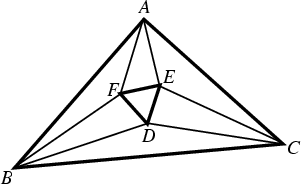The points of intersection of the adjacent Trisectors of the Angles of any Triangleare the Vertices of an Equilateral Triangleknown as Morley's Triangle. Taylor and Marr (1914) give two geometric proofs and one trigonometric proof.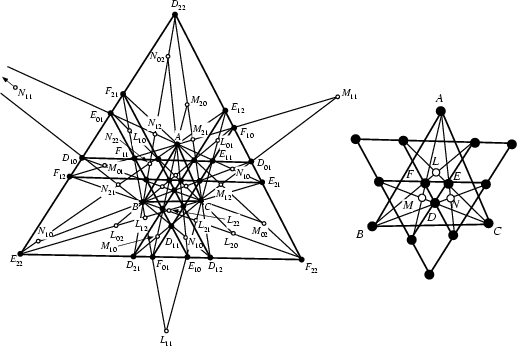A generalization of Morley's Theorem was discovered by Morley in 1900 but first published by Taylor and Marr (1914). Each Angle of a Trianglehas six trisectors, since each interior angle trisector has two associated lines making angles of 120° with it. The generalization of Morley's theorem states that these trisectors intersect in 27 points (denoted,,, for, 1, 2) which lie six by six on nine lines. Furthermore, these lines are in three triples of Parallel lines, (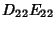,,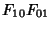), (,,), and (,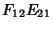,), making Angles of 60° with one another (Taylor and Marr 1914, Johnson 1929, p. 254).Let,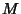, andbe the other trisector-trisector intersections, and let the 27 points,,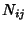for, 1, 2 be the Isogonal Conjugates of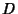,, and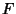. Then these points lie 6 by 6 on 9 Conics through. In addition, these Conics meet 3 by 3 on the Circumcircle, and the three meeting points form an Equilateral Triangle whose sides are Parallel to those of.

References

Coxeter, H. S. M. and Greitzer, S. L. Geometry Revisited. Washington, DC: Math. Assoc. Amer., pp. 47-50, 1967.

Gardner, M. Martin Gardner's New Mathematical Diversions from Scientific American. New York: Simon and Schuster, pp. 198 and 206, 1966.

Honsberger, R. Morley's Theorem.'' Ch. 8 in Mathematical Gems I. Washington, DC: Math. Assoc. Amer., pp. 92-98, 1973.

Johnson, R. A. Modern Geometry: An Elementary Treatise on the Geometry of the Triangle and the Circle. Boston, MA: Houghton Mifflin, pp. 253-256, 1929.

Kimberling, C. Hofstadter Points.'' Nieuw Arch. Wiskunder 12, 109-114, 1994.

Marr, W. L. Morley's Trisection Theorem: An Extension and Its Relation to the Circles of Apollonius.'' Proc. Edinburgh Math. Soc. 32, 136-150, 1914.

Oakley, C. O. and Baker, J. C. The Morley Trisector Theorem.'' Amer. Math. Monthly 85, 737-745, 1978.

Pappas, T. Trisecting & the Equilateral Triangle.'' The Joy of Mathematics. San Carlos, CA: Wide World Publ./Tetra, p. 174, 1989.

Taylor, F. G. The Relation of Morley's Theorem to the Hessian Axis and Circumcentre.'' Proc. Edinburgh Math. Soc. 32, 132-135, 1914.

Taylor, F. G. and Marr, W. L. The Six Trisectors of Each of the Angles of a Triangle.'' Proc. Edinburgh Math. Soc. 32, 119-131, 1914.